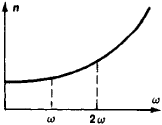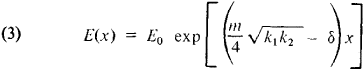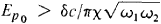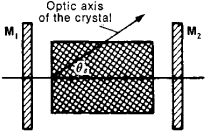# Optical Parametric Oscillator

Also found in: Acronyms, Wikipedia.

## optical parametric oscillator

[′äp·tə·kəl ¦par·ə¦me·trik ′äs·ə‚lād·ər]
(optics)
A device, employing a nonlinear dielectric, which when pumped by a laser can generate coherent light whose wavelength can be varied continuously over a wide range.

## Optical Parametric Oscillator

a source of coherent optical radiation. The basic element of an optical parametric oscillator is a nonlinear crystal in which a high-power light wave of fixed frequency parametrically excites light waves of a lower frequency. The frequencies of the parametrically excited waves are determined by the dispersion of the light in the crystal. By changing the dispersion of the medium, that is, the value of n, the frequency of the waves radiated by the optical parametric oscillator can be controlled.

Optical parametric oscillators were proposed in 1962 by S. A. Akhmanov and R. V. Khokhlov of the USSR. The first such generators were constructed in 1965 by Giordmaine and Miller in the USA and somewhat later by Akhmanov, Khokhlov, and co-workers. When a high-intensity light wave (the pump wave) propagates in a crystal, it modulates the crystal’s dielectric constant ∊. The electric field of the pump wave is

Ep = Ep0 sin(ωpt - kpx + φp

where kp = ωp/vp is the wave number, φp is the initial phase, t is time, and x is the coordinate of a point in the crystal. The dielectric constant ∊ varies in accordance with the law for a traveling wave: ∊ = ∊0[1 + m sin (ωp t + kp x + φp], where

m = 4πXEp0/∊0

is called the depth of modulation of the dielectric constant, in which X is a quantity that characterizes the nonlinear properties of the crystal.

At the entrance face (x= 0) of the crystal in which the dielectric constant ∊ is varying with time, electromagnetic oscillations may be excited at the frequencies ω1 and ω2 and the phases φ1 and φ2. The relationships between these quantities are given by the equations ωι + ω2 = ωp and φ1 + φ2 = φp, which are like the equations for the parametric excitation of oscillations in a two-circuit system. The oscillations at the frequenciesFigure 1. Relationship between the index of refraction η and the wave frequency ω for normal dispersion

ω1 and ω2 are propagated inside the crystal as two light waves. The pump wave imparts energy to the waves along the entire propagation path if the following relationship between the phases holds:

(1) φp (x) = φ1 (x) + φ2 (x)+ π/2

This corresponds to the conditions for phase matching

(2) k1 + k2 = kpFigure 2. (a) The phase-matching condition in a nonlinear crystal, (b) change in length of the wave vector kp of the extraordinary wave and k1 and k2 of the ordinary generated waves when the crystal is rotated, (c) dependence of the frequencies ω1 and ω2 of the generated waves on θ; (θ) angle between the optic axis of the crystal and the pump beam, (θx) phase-matching direction

Equation (2) indicates that the wave vectors kp of the pump wave and k1 and k2 of the excited waves form a closed triangle. It follows from (2) that the condition for the crystal’s indexes of refraction at the frequencies ωp, ω1, and ω2 is n (ωp) ≥ n (ω2) + [n(ω1) – n (ω2)]ω1p

With phase matching, the amplitudes of the excited waves continuously increase as the waves propagate in the crystal:where δ is the attenuation coefficient of the waves in the ordinary, or linear, medium. Clearly, parametric excitation occurs if the pump field exceeds the threshold:In a medium having normal dispersion, where the index of refraction n increases with the frequency ω, phase-matched interaction of the waves is unrealizable (Figure 1). In anisotropic crystals, however, where two types of waves, ordinary and extraordinary, can propagate, the phase-matching condition can be fulfilled if not only the frequency dependence of the index of refraction but also the index’s dependence on the polarization of the wave and on the direction of propagation is made use of. For example, in a negative uniaxial crystal the index of refraction of the ordinary wave n0 is greater than the index of refraction of the extraordinary wave ne, which depends on the direction of propagation of the wave with respect to the crystal’s optic axis. If the wave vectors are parallel to one another, the phase-matching condition is satisfied in the specific direction for which

(4)

2ne (ωp, θs) = no (ω1) + no (ωp ω1)

2ne (ωp, θs) = no (ω2) + no (ωpω2)

The angle θs with respect to the optic axis is called the phase-matching angle and is a function of the pump frequency and the frequency of one of the excited waves. By varying the direction of propagation of the pump wave relative to the optic axis through rotation of the crystal, the frequency of the optical parametric oscillator can be tuned smoothly (Figure 2). Other methods of frequency tuning exist; they involve the dependence of the index of refraction n on such factors as the temperature and an external electric field.

To increase the power of the optical parametric oscillator, the crystal is positioned inside an open resonator so that the waves traverse the crystal many times during the action of the pump —that is, the effective length of the crystal is increased (Figure 3). The frequency tuning of such a resonator-type optical parametric oscillator proceeds in small steps determined by the difference between the frequencies corresponding to the longitudinal modes of the resonator. Smooth tuning can be obtained by combining rotation of the crystal with variation of the resonator parameters.

Optical parametric oscillators are manufactured in many countries. The radiation of a laser (pulsed and continuous types) or of the laser’s optical harmonics serves as the pump source. Existing parametric generators cover the wavelength range from 0.5 to 4 microns. Oscillators are being developed that are tunable in the wavelength region of 10 to 15 microns. Some oscillators provide frequency tuning within 10 percent of ωp. The unique characteristics of optical parametric oscillators—coherence ofFigure 3. A nonlinear crystal positioned in an optical resonator: (M1) and (M2) mirrors forming the resonator

frequency tuning—make such a generator a basic instrument for radiation, narrowness of spectral lines, high power, and smooth spectroscopic research (for example, time-resolved spectroscopy). These characteristics also permit optical parametric oscillators to be used for selective action on a substance, especially on biological objects.

### REFERENCES

Akhmancv, S. A., and R. V. Khokhlov. “Parametricheskie usiliteli i generatory sveta.” Uspekhi fizicheskikh nauk, 1966, vol. 88, issue 3, p. 439.
Iariv, A. Kvantovaia elektronika i nelineinaia optika. Moscow, 1973. (Translated from English.)

A. P. SUKHORUKOV

References in periodicals archive ?
Contract notice: 162/LF Ti Sapphire Lasers and Optical Parametric Oscillator (OPO).
Widely tunable mid-infrared lasers, such as external cavity quantum cascade lasers (EC-QCL) and optical parametric oscillators (OPO), enable the analysis of several gas components, instead of the previously available two simultaneous components.
The topics include oxide laser crystals doped with rare earth and transition metal ions, nonlinear crystals for solid-state lasers, operation regimes for solid-state lasers, neodymium-doped yttrium aluminum garnet and neodymium-doped yttrium orthovanadate, microchip lasers, mid-infrared optical parametric oscillators, surgical solid-state lasers and their clinical applications, and environmental applications of solid-state lasers.
From then until 2010 when he moved to Austin, he was in Barcelona studying lasers and optical parametric oscillators, pump laser beams that can change photons to different wavelengths.
Now in its second edition, this manual on tunable lasers has been expanded to include recent developments over the last decade such as optical parametric oscillators, solid-state dye lasers, interferometric imaging and large free-electron lasers.
In this method two optical parametric oscillators (OPOs) are triggered with the same source.
From then until 2010 when he moved to Austin, he was a research assistant at the Institute of Photonic Sciences (ICFO) in Barcelona, Spain, studying lasers and optical parametric oscillators, pump laser beams that can change photons to different wavelengths.
It is ideal for scientific holography and high-resolution spectroscopy applications, and for pumping Ti:Sapphire lasers and optical parametric oscillators (OPO).

Site: Follow: Share:
Open / Close